Publicité

# Data Science - Part III - EDA & Model Selection

23 Feb 2015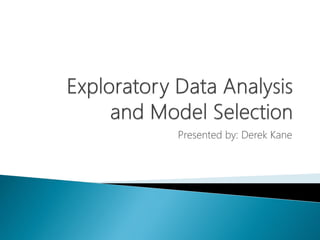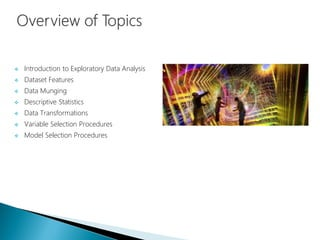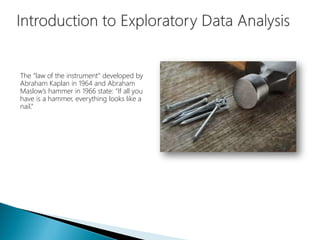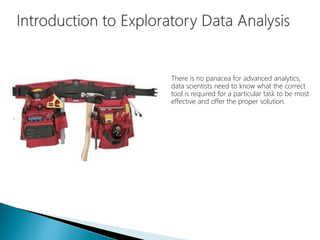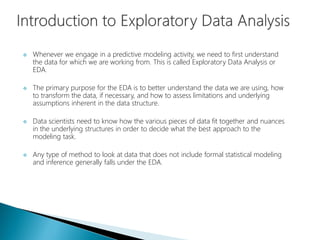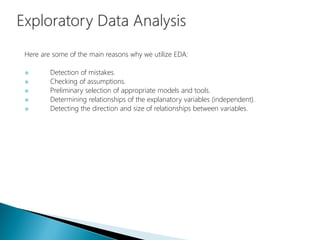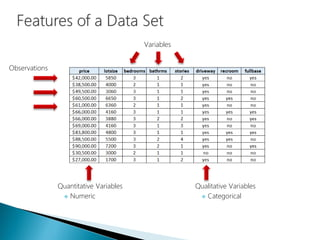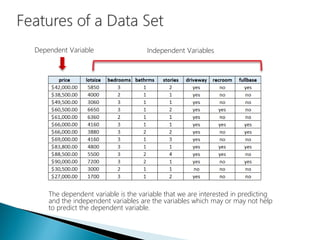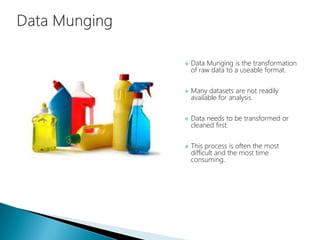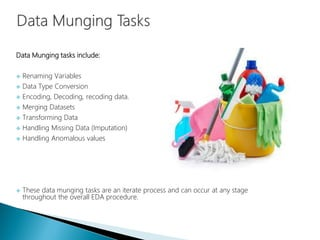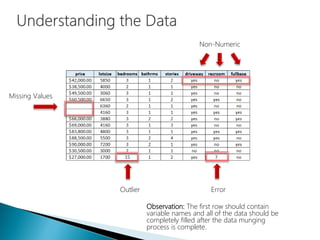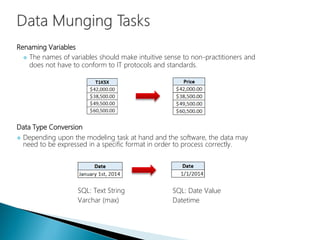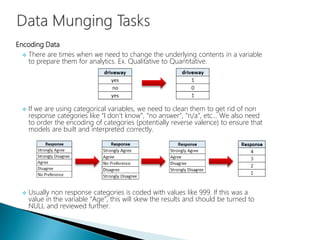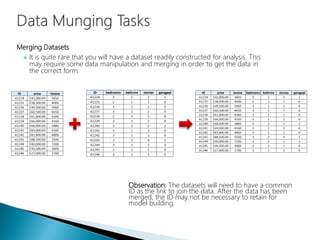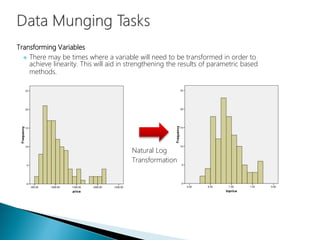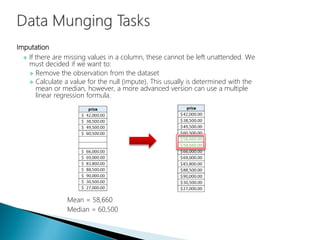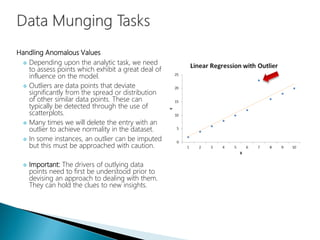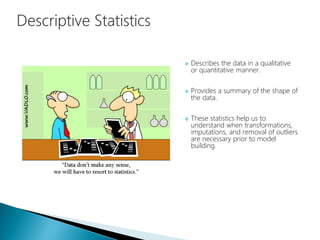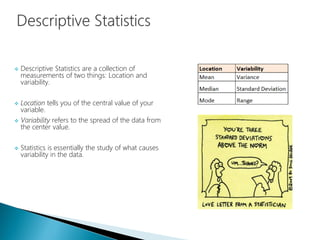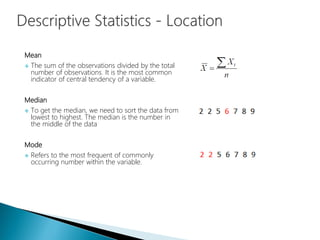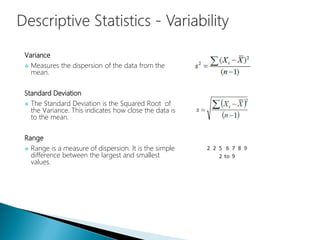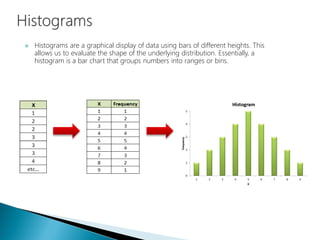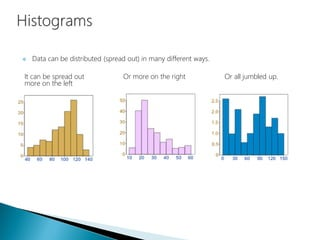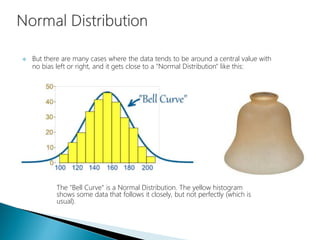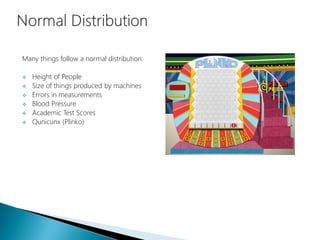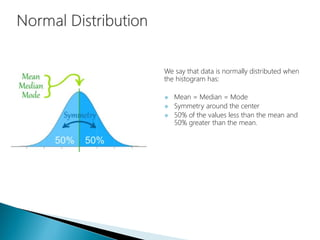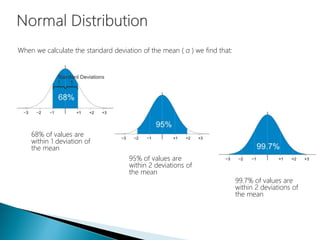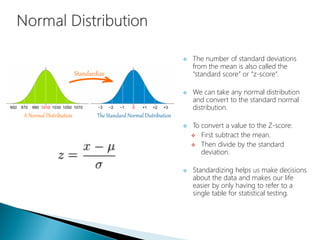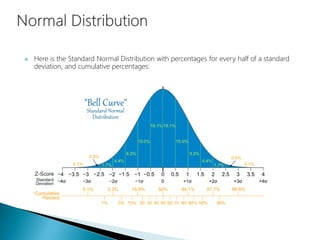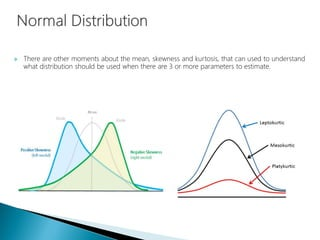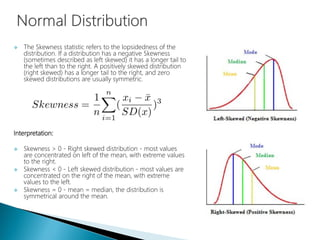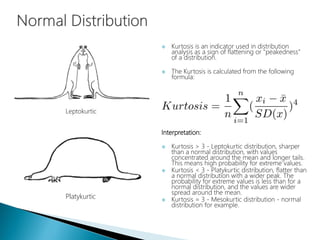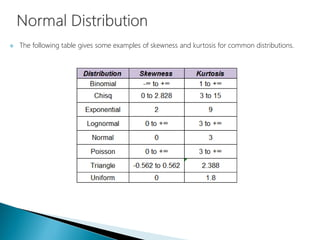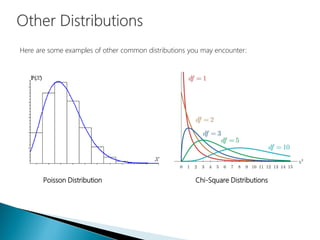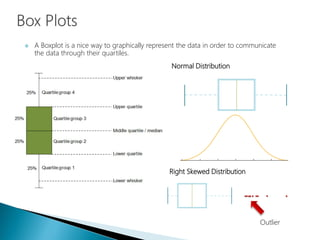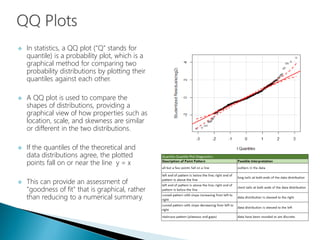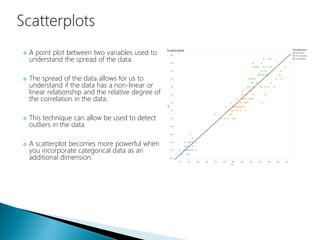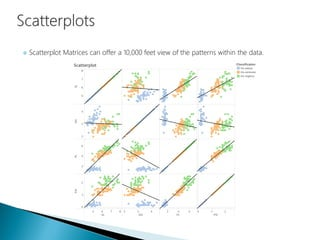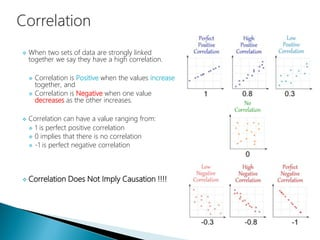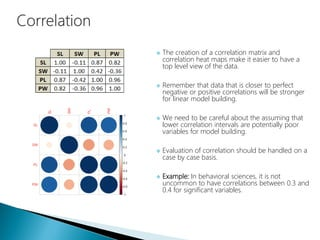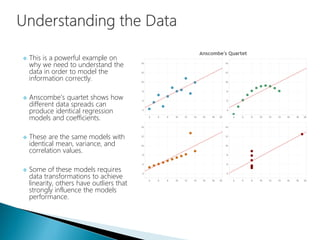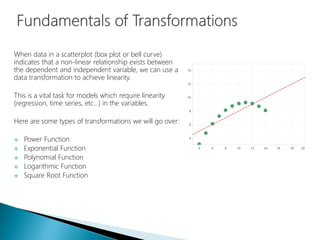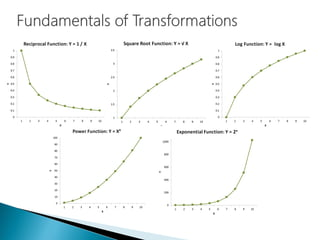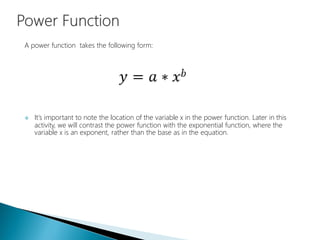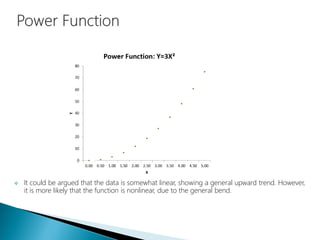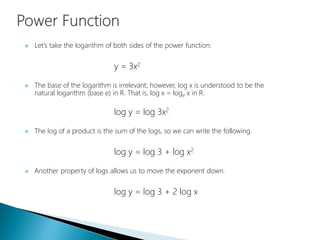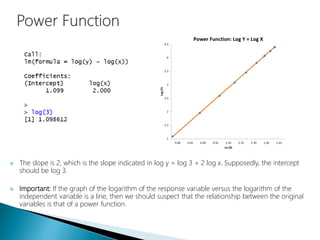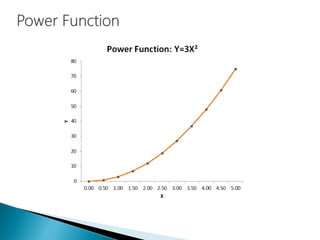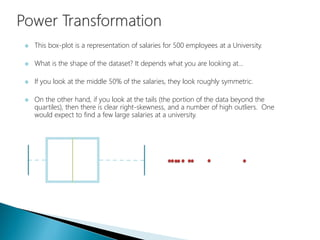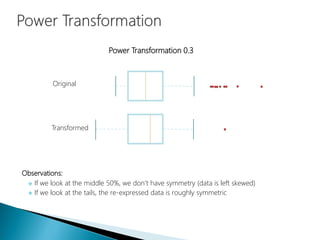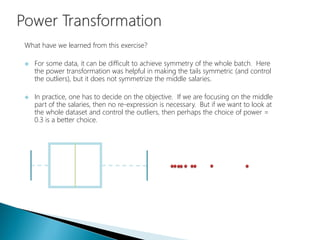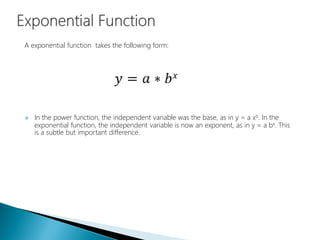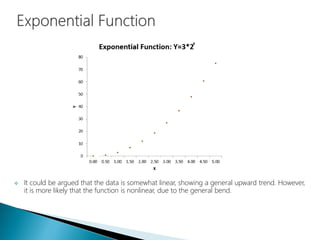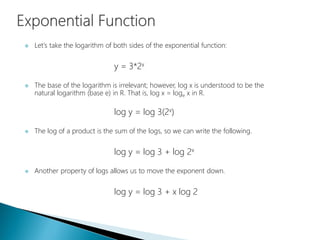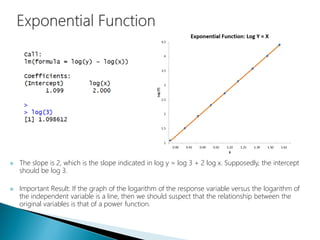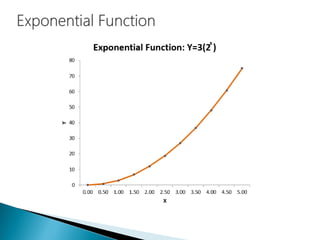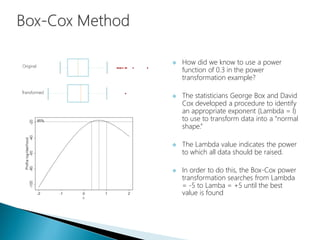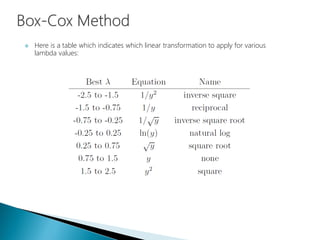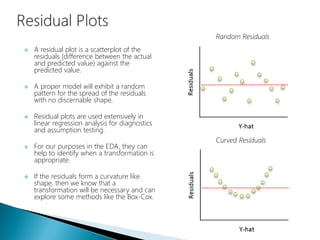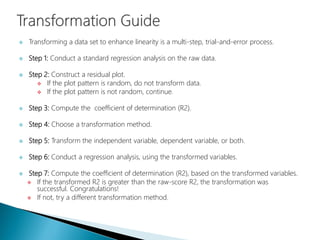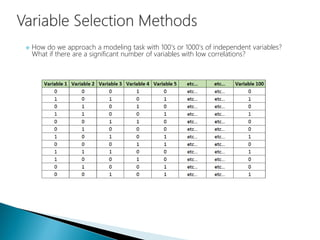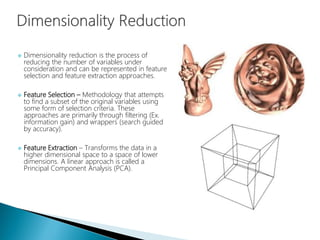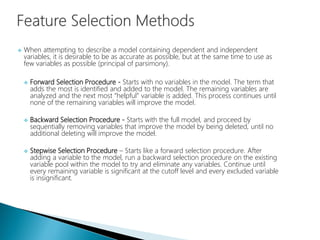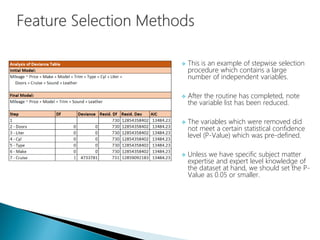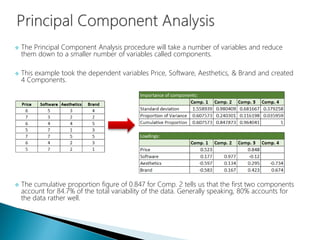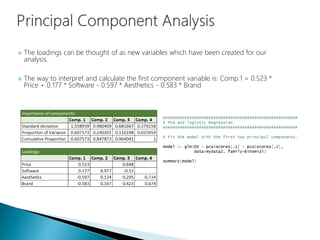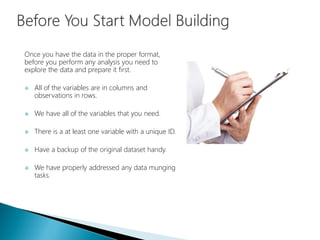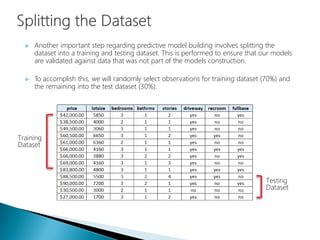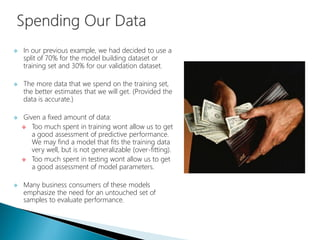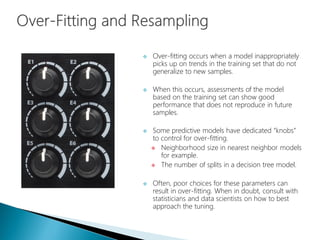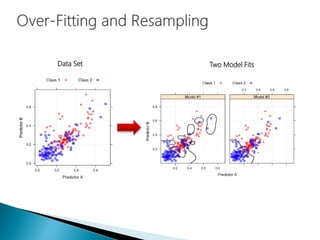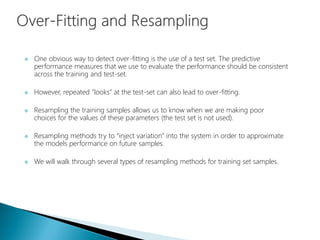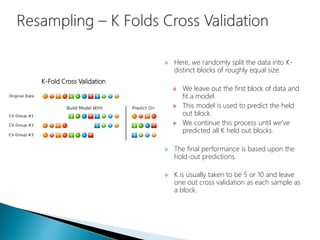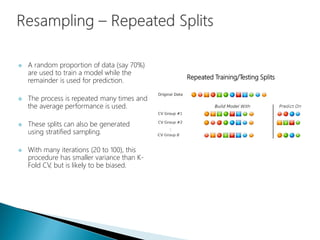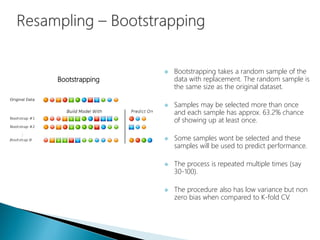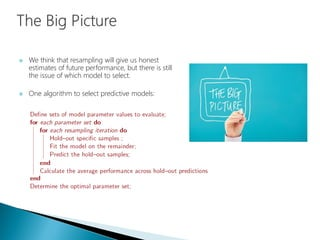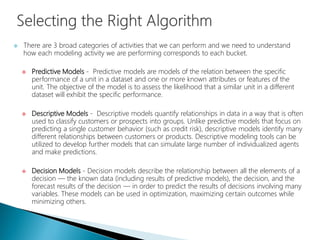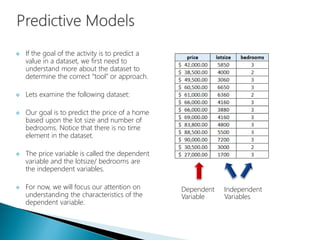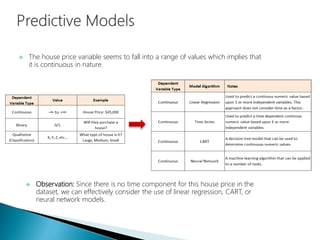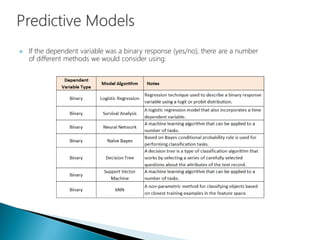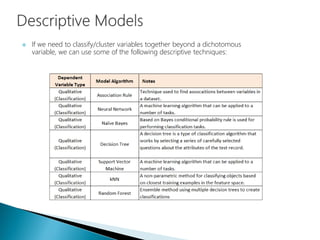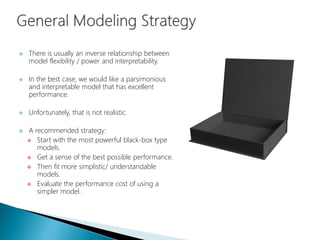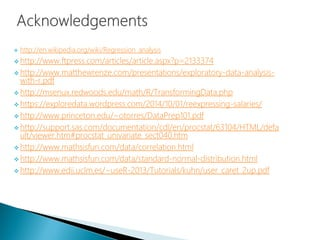1 sur 85
Publicité

### Data Science - Part III - EDA & Model Selection

1. Presented by: Derek Kane
2.  Introduction to Exploratory Data Analysis  Dataset Features  Data Munging  Descriptive Statistics  Data Transformations  Variable Selection Procedures  Model Selection Procedures
3. The “law of the instrument” developed by Abraham Kaplan in 1964 and Abraham Maslow’s hammer in 1966 state: “If all you have is a hammer, everything looks like a nail.”
4. There is no panacea for advanced analytics; data scientists need to know what the correct tool is required for a particular task to be most effective and offer the proper solution.
5.  Whenever we engage in a predictive modeling activity, we need to first understand the data for which we are working from. This is called Exploratory Data Analysis or EDA.  The primary purpose for the EDA is to better understand the data we are using, how to transform the data, if necessary, and how to assess limitations and underlying assumptions inherent in the data structure.  Data scientists need to know how the various pieces of data fit together and nuances in the underlying structures in order to decide what the best approach to the modeling task.  Any type of method to look at data that does not include formal statistical modeling and inference generally falls under the EDA.
6. Here are some of the main reasons why we utilize EDA:  Detection of mistakes.  Checking of assumptions.  Preliminary selection of appropriate models and tools.  Determining relationships of the explanatory variables (independent).  Detecting the direction and size of relationships between variables.
7. Observations Variables Qualitative Variables  Categorical Quantitative Variables  Numeric
8. Dependent Variable Independent Variables The dependent variable is the variable that we are interested in predicting and the independent variables are the variables which may or may not help to predict the dependent variable.
9.  Data Munging is the transformation of raw data to a useable format.  Many datasets are not readily available for analysis.  Data needs to be transformed or cleaned first.  This process is often the most difficult and the most time consuming.
10. Data Munging tasks include:  Renaming Variables  Data Type Conversion  Encoding, Decoding, recoding data.  Merging Datasets  Transforming Data  Handling Missing Data (Imputation)  Handling Anomalous values  These data munging tasks are an iterate process and can occur at any stage throughout the overall EDA procedure.
11. Missing Values Outlier Error Non-Numeric Observation: The first row should contain variable names and all of the data should be completely filled after the data munging process is complete.
12. Renaming Variables  The names of variables should make intuitive sense to non-practitioners and does not have to conform to IT protocols and standards. Data Type Conversion  Depending upon the modeling task at hand and the software, the data may need to be expressed in a specific format in order to process correctly. SQL: Text String Varchar (max) SQL: Date Value Datetime
13. Encoding Data  There are times when we need to change the underlying contents in a variable to prepare them for analytics. Ex. Qualitative to Quantitative.  If we are using categorical variables, we need to clean them to get rid of non response categories like “I don’t know”, “no answer”, “n/a”, etc… We also need to order the encoding of categories (potentially reverse valence) to ensure that models are built and interpreted correctly.  Usually non response categories is coded with values like 999. If this was a value in the variable “Age”, this will skew the results and should be turned to NULL and reviewed further.
14. Merging Datasets  It is quite rare that you will have a dataset readily constructed for analysis. This may require some data manipulation and merging in order to get the data in the correct form. Observation: The datasets will need to have a common ID as the link to join the data. After the data has been merged, the ID may not be necessary to retain for model building.
15. Transforming Variables  There may be times where a variable will need to be transformed in order to achieve linearity. This will aid in strengthening the results of parametric based methods. Natural Log Transformation
16. Imputation  If there are missing values in a column, these cannot be left unattended. We must decided if we want to:  Remove the observation from the dataset  Calculate a value for the null (impute). This usually is determined with the mean or median, however, a more advanced version can use a multiple linear regression formula. Mean = 58,660 Median = 60,500
17. Handling Anomalous Values  Depending upon the analytic task, we need to assess points which exhibit a great deal of influence on the model.  Outliers are data points that deviate significantly from the spread or distribution of other similar data points. These can typically be detected through the use of scatterplots.  Many times we will delete the entry with an outlier to achieve normality in the dataset.  In some instances, an outlier can be imputed but this must be approached with caution.  Important: The drivers of outlying data points need to first be understood prior to devising an approach to dealing with them. They can hold the clues to new insights.
18.  Describes the data in a qualitative or quantitative manner.  Provides a summary of the shape of the data.  These statistics help us to understand when transformations, imputations, and removal of outliers are necessary prior to model building.
19.  Descriptive Statistics are a collection of measurements of two things: Location and variability.  Location tells you of the central value of your variable.  Variability refers to the spread of the data from the center value.  Statistics is essentially the study of what causes variability in the data.
20. Mean  The sum of the observations divided by the total number of observations. It is the most common indicator of central tendency of a variable. Median  To get the median, we need to sort the data from lowest to highest. The median is the number in the middle of the data Mode  Refers to the most frequent of commonly occurring number within the variable.
21. Variance  Measures the dispersion of the data from the mean. Standard Deviation  The Standard Deviation is the Squared Root of the Variance. This indicates how close the data is to the mean. Range  Range is a measure of dispersion. It is the simple difference between the largest and smallest values.
22.  Histograms are a graphical display of data using bars of different heights. This allows us to evaluate the shape of the underlying distribution. Essentially, a histogram is a bar chart that groups numbers into ranges or bins.
23.  Data can be distributed (spread out) in many different ways. It can be spread out more on the left Or more on the right Or all jumbled up.
24.  But there are many cases where the data tends to be around a central value with no bias left or right, and it gets close to a "Normal Distribution" like this: The "Bell Curve" is a Normal Distribution. The yellow histogram shows some data that follows it closely, but not perfectly (which is usual).
25. Many things follow a normal distribution:  Height of People  Size of things produced by machines  Errors in measurements  Blood Pressure  Academic Test Scores  Qunicunx (Plinko)
26. We say that data is normally distributed when the histogram has:  Mean = Median = Mode  Symmetry around the center  50% of the values less than the mean and 50% greater than the mean.
27. When we calculate the standard deviation of the mean ( σ ) we find that: 68% of values are within 1 deviation of the mean 95% of values are within 2 deviations of the mean 99.7% of values are within 2 deviations of the mean
28.  The number of standard deviations from the mean is also called the “standard score” or “z-score”.  We can take any normal distribution and convert to the standard normal distribution.  To convert a value to the Z-score:  First subtract the mean.  Then divide by the standard deviation.  Standardizing helps us make decisions about the data and makes our life easier by only having to refer to a single table for statistical testing.
29.  Here is the Standard Normal Distribution with percentages for every half of a standard deviation, and cumulative percentages:
30.  There are other moments about the mean, skewness and kurtosis, that can used to understand what distribution should be used when there are 3 or more parameters to estimate.
31.  The Skewness statistic refers to the lopsidedness of the distribution. If a distribution has a negative Skewness (sometimes described as left skewed) it has a longer tail to the left than to the right. A positively skewed distribution (right skewed) has a longer tail to the right, and zero skewed distributions are usually symmetric. Interpretation:  Skewness > 0 - Right skewed distribution - most values are concentrated on left of the mean, with extreme values to the right.  Skewness < 0 - Left skewed distribution - most values are concentrated on the right of the mean, with extreme values to the left.  Skewness = 0 - mean = median, the distribution is symmetrical around the mean.
32.  Kurtosis is an indicator used in distribution analysis as a sign of flattening or "peakedness" of a distribution.  The Kurtosis is calculated from the following formula: Interpretation:  Kurtosis > 3 - Leptokurtic distribution, sharper than a normal distribution, with values concentrated around the mean and longer tails. This means high probability for extreme values.  Kurtosis < 3 - Platykurtic distribution, flatter than a normal distribution with a wider peak. The probability for extreme values is less than for a normal distribution, and the values are wider spread around the mean.  Kurtosis = 3 - Mesokurtic distribution - normal distribution for example. Leptokurtic Platykurtic
33.  The following table gives some examples of skewness and kurtosis for common distributions.
34. Here are some examples of other common distributions you may encounter: Poisson Distribution Chi-Square Distributions
35.  A Boxplot is a nice way to graphically represent the data in order to communicate the data through their quartiles. Outlier Right Skewed Distribution Normal Distribution
36.  In statistics, a QQ plot ("Q" stands for quantile) is a probability plot, which is a graphical method for comparing two probability distributions by plotting their quantiles against each other.  A QQ plot is used to compare the shapes of distributions, providing a graphical view of how properties such as location, scale, and skewness are similar or different in the two distributions.  If the quantiles of the theoretical and data distributions agree, the plotted points fall on or near the line y = x  This can provide an assessment of "goodness of fit" that is graphical, rather than reducing to a numerical summary.
37.  A point plot between two variables used to understand the spread of the data.  The spread of the data allows for us to understand if the data has a non-linear or linear relationship and the relative degree of the correlation in the data.  This technique can allow be used to detect outliers in the data.  A scatterplot becomes more powerful when you incorporate categorical data as an additional dimension.
38.  Scatterplot Matrices can offer a 10,000 feet view of the patterns within the data.
39.  When two sets of data are strongly linked together we say they have a high correlation.  Correlation is Positive when the values increase together, and  Correlation is Negative when one value decreases as the other increases.  Correlation can have a value ranging from:  1 is perfect positive correlation  0 implies that there is no correlation  -1 is perfect negative correlation  Correlation Does Not Imply Causation !!!!
40.  The creation of a correlation matrix and correlation heat maps make it easier to have a top level view of the data.  Remember that data that is closer to perfect negative or positive correlations will be stronger for linear model building.  We need to be careful about the assuming that lower correlation intervals are potentially poor variables for model building.  Evaluation of correlation should be handled on a case by case basis.  Example: In behavioral sciences, it is not uncommon to have correlations between 0.3 and 0.4 for significant variables.
41.  This is a powerful example on why we need to understand the data in order to model the information correctly.  Anscombe’s quartet shows how different data spreads can produce identical regression models and coefficients.  These are the same models with identical mean, variance, and correlation values.  Some of these models requires data transformations to achieve linearity, others have outliers that strongly influence the models performance.
42. When data in a scatterplot (box plot or bell curve) indicates that a non-linear relationship exists between the dependent and independent variable, we can use a data transformation to achieve linearity. This is a vital task for models which require linearity (regression, time series, etc…) in the variables. Here are some types of transformations we will go over:  Power Function  Exponential Function  Polynomial Function  Logarithmic Function  Square Root Function
43. A power function takes the following form:  It's important to note the location of the variable x in the power function. Later in this activity, we will contrast the power function with the exponential function, where the variable x is an exponent, rather than the base as in the equation.
44.  It could be argued that the data is somewhat linear, showing a general upward trend. However, it is more likely that the function is nonlinear, due to the general bend.
45.  Let's take the logarithm of both sides of the power function: y = 3x2  The base of the logarithm is irrelevant; however, log x is understood to be the natural logarithm (base e) in R. That is, log x = loge x in R. log y = log 3x2  The log of a product is the sum of the logs, so we can write the following. log y = log 3 + log x2  Another property of logs allows us to move the exponent down. log y = log 3 + 2 log x
46.  The slope is 2, which is the slope indicated in log y = log 3 + 2 log x. Supposedly, the intercept should be log 3.  Important: If the graph of the logarithm of the response variable versus the logarithm of the independent variable is a line, then we should suspect that the relationship between the original variables is that of a power function.
47.  This box-plot is a representation of salaries for 500 employees at a University.  What is the shape of the dataset? It depends what you are looking at…  If you look at the middle 50% of the salaries, they look roughly symmetric.  On the other hand, if you look at the tails (the portion of the data beyond the quartiles), then there is clear right-skewness, and a number of high outliers. One would expect to find a few large salaries at a university.
48. Original Transformed Power Transformation 0.3 Observations:  If we look at the middle 50%, we don’t have symmetry (data is left skewed)  If we look at the tails, the re-expressed data is roughly symmetric
49. What have we learned from this exercise?  For some data, it can be difficult to achieve symmetry of the whole batch. Here the power transformation was helpful in making the tails symmetric (and control the outliers), but it does not symmetrize the middle salaries.  In practice, one has to decide on the objective. If we are focusing on the middle part of the salaries, then no re-expression is necessary. But if we want to look at the whole dataset and control the outliers, then perhaps the choice of power = 0.3 is a better choice.
50. A exponential function takes the following form:  In the power function, the independent variable was the base, as in y = a xb. In the exponential function, the independent variable is now an exponent, as in y = a bx. This is a subtle but important difference.
51.  It could be argued that the data is somewhat linear, showing a general upward trend. However, it is more likely that the function is nonlinear, due to the general bend.
52.  Let's take the logarithm of both sides of the exponential function: y = 3*2x  The base of the logarithm is irrelevant; however, log x is understood to be the natural logarithm (base e) in R. That is, log x = loge x in R. log y = log 3(2x)  The log of a product is the sum of the logs, so we can write the following. log y = log 3 + log 2x  Another property of logs allows us to move the exponent down. log y = log 3 + x log 2
53.  The slope is 2, which is the slope indicated in log y = log 3 + 2 log x. Supposedly, the intercept should be log 3.  Important Result: If the graph of the logarithm of the response variable versus the logarithm of the independent variable is a line, then we should suspect that the relationship between the original variables is that of a power function.
54.  How did we know to use a power function of 0.3 in the power transformation example?  The statisticians George Box and David Cox developed a procedure to identify an appropriate exponent (Lambda = l) to use to transform data into a “normal shape.”  The Lambda value indicates the power to which all data should be raised.  In order to do this, the Box-Cox power transformation searches from Lambda = -5 to Lamba = +5 until the best value is found
55.  Here is a table which indicates which linear transformation to apply for various lambda values:
56.  A residual plot is a scatterplot of the residuals (difference between the actual and predicted value) against the predicted value.  A proper model will exhibit a random pattern for the spread of the residuals with no discernable shape.  Residual plots are used extensively in linear regression analysis for diagnostics and assumption testing.  For our purposes in the EDA, they can help to identify when a transformation is appropriate.  If the residuals form a curvature like shape, then we know that a transformation will be necessary and can explore some methods like the Box-Cox. Random Residuals Curved Residuals
57.  Transforming a data set to enhance linearity is a multi-step, trial-and-error process.  Step 1: Conduct a standard regression analysis on the raw data.  Step 2: Construct a residual plot.  If the plot pattern is random, do not transform data.  If the plot pattern is not random, continue.  Step 3: Compute the coefficient of determination (R2).  Step 4: Choose a transformation method.  Step 5: Transform the independent variable, dependent variable, or both.  Step 6: Conduct a regression analysis, using the transformed variables.  Step 7: Compute the coefficient of determination (R2), based on the transformed variables.  If the transformed R2 is greater than the raw-score R2, the transformation was successful. Congratulations!  If not, try a different transformation method.
58.  How do we approach a modeling task with 100’s or 1000’s of independent variables? What if there are a significant number of variables with low correlations?
59.  Dimensionality reduction is the process of reducing the number of variables under consideration and can be represented in feature selection and feature extraction approaches.  Feature Selection – Methodology that attempts to find a subset of the original variables using some form of selection criteria. These approaches are primarily through filtering (Ex. information gain) and wrappers (search guided by accuracy).  Feature Extraction – Transforms the data in a higher dimensional space to a space of lower dimensions. A linear approach is called a Principal Component Analysis (PCA).
60.  When attempting to describe a model containing dependent and independent variables, it is desirable to be as accurate as possible, but at the same time to use as few variables as possible (principal of parsimony).  Forward Selection Procedure - Starts with no variables in the model. The term that adds the most is identified and added to the model. The remaining variables are analyzed and the next most “helpful” variable is added. This process continues until none of the remaining variables will improve the model.  Backward Selection Procedure - Starts with the full model, and proceed by sequentially removing variables that improve the model by being deleted, until no additional deleting will improve the model.  Stepwise Selection Procedure – Starts like a forward selection procedure. After adding a variable to the model, run a backward selection procedure on the existing variable pool within the model to try and eliminate any variables. Continue until every remaining variable is significant at the cutoff level and every excluded variable is insignificant.
61.  This is an example of stepwise selection procedure which contains a large number of independent variables.  After the routine has completed, note the variable list has been reduced.  The variables which were removed did not meet a certain statistical confidence level (P-Value) which was pre-defined.  Unless we have specific subject matter expertise and expert level knowledge of the dataset at hand, we should set the P- Value as 0.05 or smaller.
62.  The Principal Component Analysis procedure will take a number of variables and reduce them down to a smaller number of variables called components.  This example took the dependent variables Price, Software, Aesthetics, & Brand and created 4 Components.  The cumulative proportion figure of 0.847 for Comp. 2 tells us that the first two components account for 84.7% of the total variability of the data. Generally speaking, 80% accounts for the data rather well.
63.  The loadings can be thought of as new variables which have been created for our analysis.  The way to interpret and calculate the first component variable is: Comp.1 = 0.523 * Price + 0.177 * Software - 0.597 * Aesthetics - 0.583 * Brand
64. Once you have the data in the proper format, before you perform any analysis you need to explore the data and prepare it first.  All of the variables are in columns and observations in rows.  We have all of the variables that you need.  There is a at least one variable with a unique ID.  Have a backup of the original dataset handy.  We have properly addressed any data munging tasks.
65.  Another important step regarding predictive model building involves splitting the dataset into a training and testing dataset. This is performed to ensure that our models are validated against data that was not part of the models construction.  To accomplish this, we will randomly select observations for training dataset (70%) and the remaining into the test dataset (30%). Training Dataset Testing Dataset
66.  In our previous example, we had decided to use a split of 70% for the model building dataset or training set and 30% for our validation dataset.  The more data that we spend on the training set, the better estimates that we will get. (Provided the data is accurate.)  Given a fixed amount of data:  Too much spent in training wont allow us to get a good assessment of predictive performance. We may find a model that fits the training data very well, but is not generalizable (over-fitting).  Too much spent in testing wont allow us to get a good assessment of model parameters.  Many business consumers of these models emphasize the need for an untouched set of samples to evaluate performance.
67.  Over-fitting occurs when a model inappropriately picks up on trends in the training set that do not generalize to new samples.  When this occurs, assessments of the model based on the training set can show good performance that does not reproduce in future samples.  Some predictive models have dedicated “knobs” to control for over-fitting.  Neighborhood size in nearest neighbor models for example.  The number of splits in a decision tree model.  Often, poor choices for these parameters can result in over-fitting. When in doubt, consult with statisticians and data scientists on how to best approach the tuning.
68. Data Set Two Model Fits
69.  One obvious way to detect over-fitting is the use of a test set. The predictive performance measures that we use to evaluate the performance should be consistent across the training and test-set.  However, repeated “looks” at the test-set can also lead to over-fitting.  Resampling the training samples allows us to know when we are making poor choices for the values of these parameters (the test set is not used).  Resampling methods try to “inject variation” into the system in order to approximate the models performance on future samples.  We will walk through several types of resampling methods for training set samples.
70.  Here, we randomly split the data into K- distinct blocks of roughly equal size.  We leave out the first block of data and fit a model.  This model is used to predict the held out block.  We continue this process until we’ve predicted all K held out blocks.  The final performance is based upon the hold-out predictions.  K is usually taken to be 5 or 10 and leave one out cross validation as each sample as a block. K-Fold Cross Validation
71.  A random proportion of data (say 70%) are used to train a model while the remainder is used for prediction.  The process is repeated many times and the average performance is used.  These splits can also be generated using stratified sampling.  With many iterations (20 to 100), this procedure has smaller variance than K- Fold CV, but is likely to be biased. Repeated Training/Testing Splits
72.  Bootstrapping takes a random sample of the data with replacement. The random sample is the same size as the original dataset.  Samples may be selected more than once and each sample has approx. 63.2% chance of showing up at least once.  Some samples wont be selected and these samples will be used to predict performance.  The process is repeated multiple times (say 30-100).  The procedure also has low variance but non zero bias when compared to K-fold CV. Bootstrapping
73.  We think that resampling will give us honest estimates of future performance, but there is still the issue of which model to select.  One algorithm to select predictive models:
74.  There are 3 broad categories of activities that we can perform and we need to understand how each modeling activity we are performing corresponds to each bucket.  Predictive Models - Predictive models are models of the relation between the specific performance of a unit in a dataset and one or more known attributes or features of the unit. The objective of the model is to assess the likelihood that a similar unit in a different dataset will exhibit the specific performance.  Descriptive Models - Descriptive models quantify relationships in data in a way that is often used to classify customers or prospects into groups. Unlike predictive models that focus on predicting a single customer behavior (such as credit risk), descriptive models identify many different relationships between customers or products. Descriptive modeling tools can be utilized to develop further models that can simulate large number of individualized agents and make predictions.  Decision Models - Decision models describe the relationship between all the elements of a decision — the known data (including results of predictive models), the decision, and the forecast results of the decision — in order to predict the results of decisions involving many variables. These models can be used in optimization, maximizing certain outcomes while minimizing others.
75.  If the goal of the activity is to predict a value in a dataset, we first need to understand more about the dataset to determine the correct “tool” or approach.  Lets examine the following dataset:  Our goal is to predict the price of a home based upon the lot size and number of bedrooms. Notice that there is no time element in the dataset.  The price variable is called the dependent variable and the lotsize/ bedrooms are the independent variables.  For now, we will focus our attention on understanding the characteristics of the dependent variable. Dependent Variable Independent Variables
76.  The house price variable seems to fall into a range of values which implies that it is continuous in nature.  Observation: Since there is no time component for this house price in the dataset, we can effectively consider the use of linear regression, CART, or neural network models.
77.  If the dependent variable was a binary response (yes/no), there are a number of different methods we would consider using:
78.  If we need to classify/cluster variables together beyond a dichotomous variable, we can use some of the following descriptive techniques:
79.  There is usually an inverse relationship between model flexibility / power and interpretability.  In the best case, we would like a parsimonious and interpretable model that has excellent performance.  Unfortunately, that is not realistic.  A recommended strategy:  Start with the most powerful black-box type models.  Get a sense of the best possible performance.  Then fit more simplistic/ understandable models.  Evaluate the performance cost of using a simpler model.
80.  Reside in Wayne, Illinois  Active Semi-Professional Classical Musician (Bassoon).  Married my wife on 10/10/10 and been together for 10 years.  Pet Yorkshire Terrier / Toy Poodle named Brunzie.  Pet Maine Coons’ named Maximus Power and Nemesis Gul du Cat.  Enjoy Cooking, Hiking, Cycling, Kayaking, and Astronomy.  Self proclaimed Data Nerd and Technology Lover.
81.  http://en.wikipedia.org/wiki/Regression_analysis  http://www.ftpress.com/articles/article.aspx?p=2133374  http://www.matthewrenze.com/presentations/exploratory-data-analysis- with-r.pdf  http://msenux.redwoods.edu/math/R/TransformingData.php  https://exploredata.wordpress.com/2014/10/01/reexpressing-salaries/  http://www.princeton.edu/~otorres/DataPrep101.pdf  http://support.sas.com/documentation/cdl/en/procstat/63104/HTML/defa ult/viewer.htm#procstat_univariate_sect040.htm  http://www.mathsisfun.com/data/correlation.html  http://www.mathsisfun.com/data/standard-normal-distribution.html  http://www.edii.uclm.es/~useR-2013/Tutorials/kuhn/user_caret_2up.pdf
Publicité